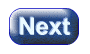created 08/26/97; revised 08/13/00; 10/07/00; 07/25/03

# CHAPTER 9 — The Angle Between Two Vectors

The previous chapter discussed some uses of the dot product: computing the length of a vector, and determining if two vectors are perpendicular:

• Length: dot product of vector with itself = length2
• Orthogonality: dot product of two perpendicular vectors = 0

In the first case, the angle between the vector and itself is 0°. In the second case, the angle between the two vectors is 90°.

### QUESTION 1:Does the angle between two vectors have something to do with the dot product?